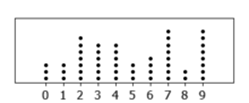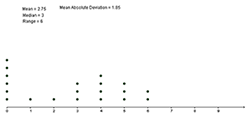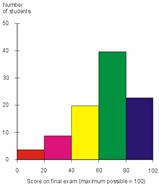Dot Plot

My Definition

Key Characteristics

The following are true of a dot plot:

• The data may be numerical or categorical.

• Dots, indicating frequencies, are placed vertically above a line labeled with categories or numerical values.

• The dots may represent one count or more if so noted.

• Dot plots are best for organizing a small set of data with a relatively small range.

• A dot plot of numerical data shows the range, outliers, shape, and spread of the data.

Example

A sample dot plot is shown below.

Dotplot of Random ValuesRandom Values

If a number is deleted the interactive will need to be restarted. Numbers can only be replaced.

Dot Plot ActivityNon-example

Scores on Final Exams is an example of a histogram.

Scores on Final ExamTEKS: 3(8)(A), 3(8)(B), 4(9)(A), 4(9)(B), 5(9)(A), 5(9)(C), 6(12)(A), 6(13)(A), 7(6)(G),
7(12)(A)